# Meta Learning - Gradient Descent as LSTM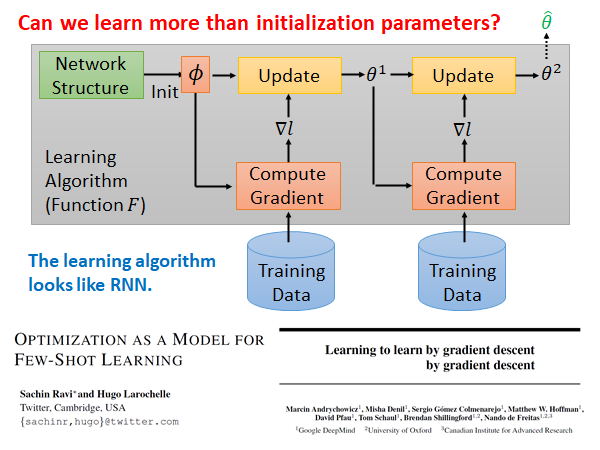Optimization as a Model for Few-Shot Learning | OpenReview

Sachin Ravi, Hugo Larochelle

Marcin Andrychowicz, Misha Denil, Sergio Gomez, Matthew W. Hoffman, David Pfau, Tom Schaul, Brendan Shillingford, Nando de Freitas

## Review: RNN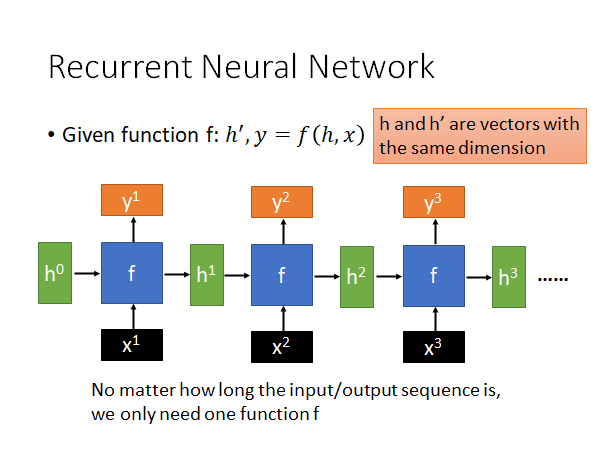RNN就是一个function f，这个函数吃h,x 吐出 h’,y ，每个step 会有一个x（训练样本数据）作为input，还有一个初始的memory 的值 $h_0$ 作为input，这个初始参数有时候是人手动设置的，有时候是可以让模型learn 出来的，然后输出一个y和一个 $h^1$ 。到下一个step，它吃上一个step 得到的 $h^1$ 和新的x，也是同样的输出。需要注意的是，h的维度都是一致的，这样同一个f 才能吃前一个step 得到h 。这个过程不断重复，就是RNN。• $c$ 变化较慢，通常就是把某个向量加到上一个 $c^{t-1}$ 上就得到了新的 $c^t$ ，这个 $c^t$ 就是LSTM 中memory cell 存储的值，由于这个值变化很慢，所以LSTM 可以记住时间比较久的数据
• $h$ 变化较快， $h^{t-1}$$h^t$ 的变化是很大的

## Review: LSTM$c^{t-1}$ 是memory 记忆单元，把x和h拼在一起乘上一个权重矩阵W，再通过一个tanh 函数得到input z，z是一个向量。同样的x和h拼接后乘上对应的权重矩阵得到对应向量input gate $z^i$ ，forget gate $z^f$ ，output gate $z^o$ ，接下来：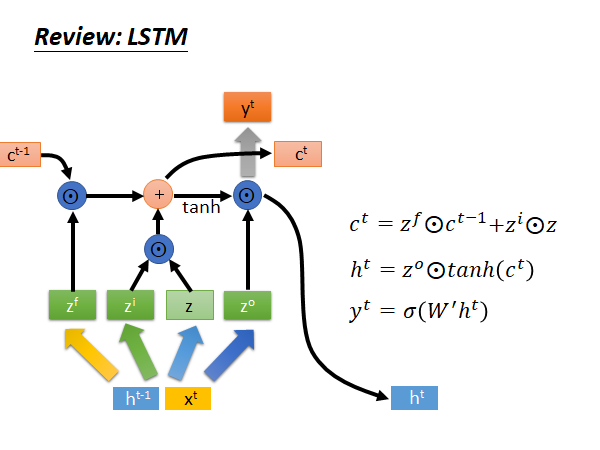$z^f \cdot c^{t-1}$ 决定是否保留上个memory， $z^i \cdot z$ 决定是否把现在的input 存到memory；

$W'$ 乘上新的 $h^t$ ，再通过一个sigmoid function 得到当前step 的output $y^t$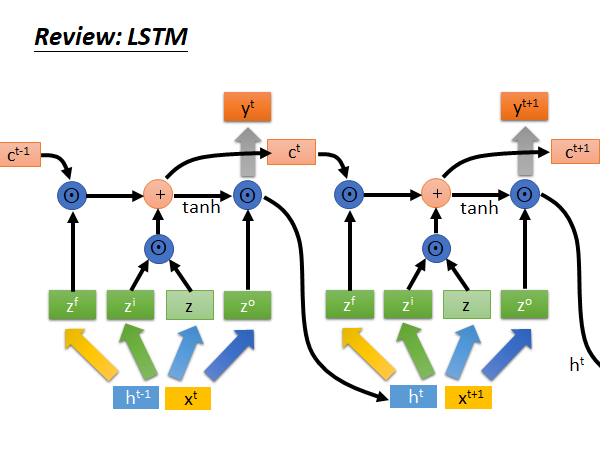## LSTM similar to gradient descent based algorithm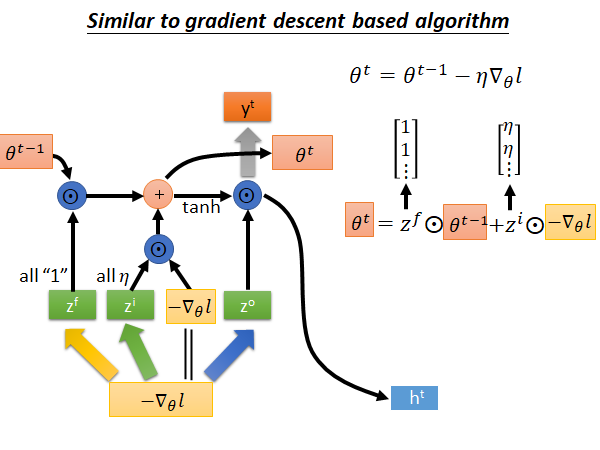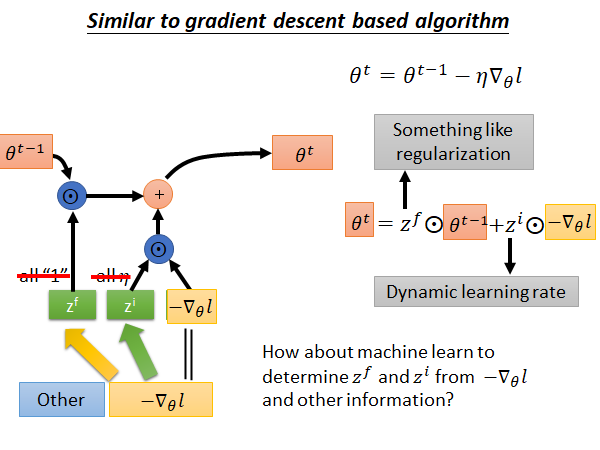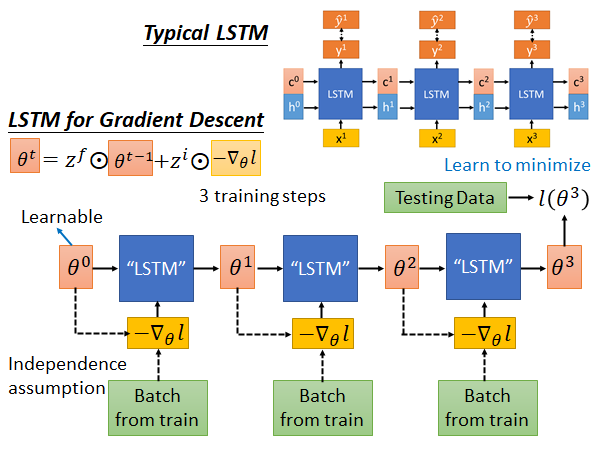Typical LSTM 就是input x ，output c 和 h，每个step 会output 一个y ，希望y 和label 越接近越好。

Gradient Descent 的LSTM是这样：我们先sample 一个初始参数θ ，然后sample 一个batch 的data ，根据这一组data 算出一个gradient $\nabla_\theta l$ ，把负的gradient input 到LSTM 中进行训练，这个LSTM 的参数过去是人设死的，我们现在让参数在Meta Learning 的架构下被硬learn 出来。上述的这个update 参数的公式就是：

$\theta^t = z^f \cdot \theta^{t-1} + z^i \cdot -\nabla_\theta l$

$z^f$ $z^i$ 以前是人设死的，现在LSTM 可以自动把它学出来。

## Real Implementation

LSTM 的memory 就是要训练的network 的参数，这些参数动辄就是十万百万级别的，难道要开十万百万个cell 吗？平常我们开上千个cell 就会train 很久，所以这样是train不起来的。在实际的实现上，我们做了一个非常大的简化：我们所learn 的LSTM 只有一个cell 而已，它只处理一个参数，所有的参数都公用一个LSTM。所以就算你有百万个参数，都是使用这同一个LSTM 来处理。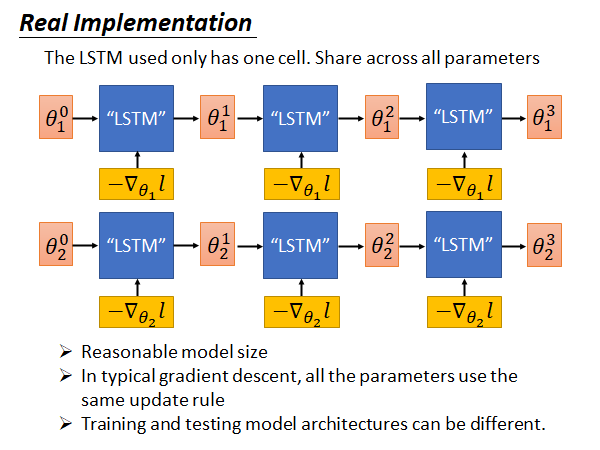• 在模型规模上问题上比较容易实现
• 训练和测试的模型架构可以是不一样的，而之前讲的MAML 需要保证训练任务和测试任务使用的model architecture 相同

## Experimental Results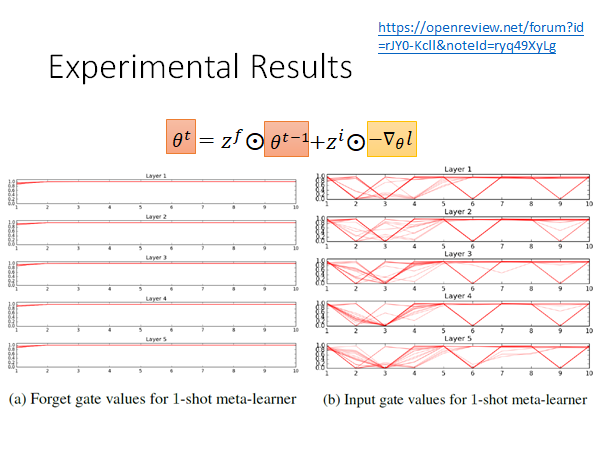https://openreview.net/forum?id=rJY0-Kcll&noteId=ryq49XyLg

## LSTM for Gradient Descent (v2)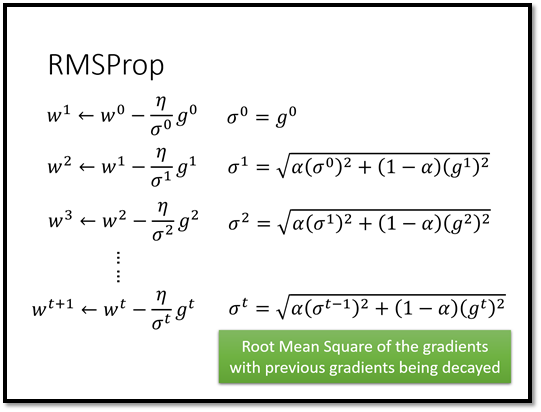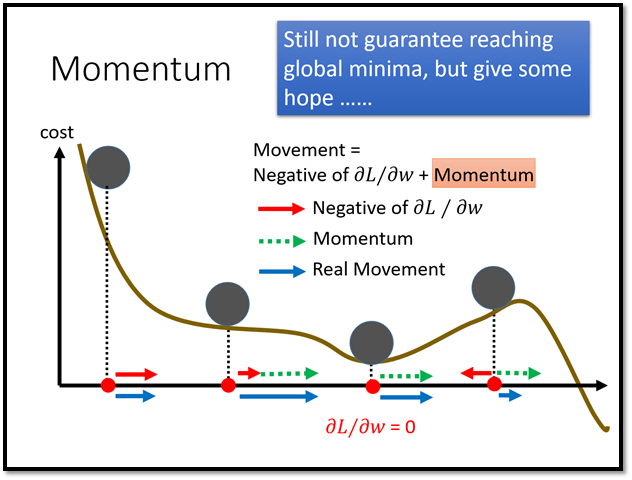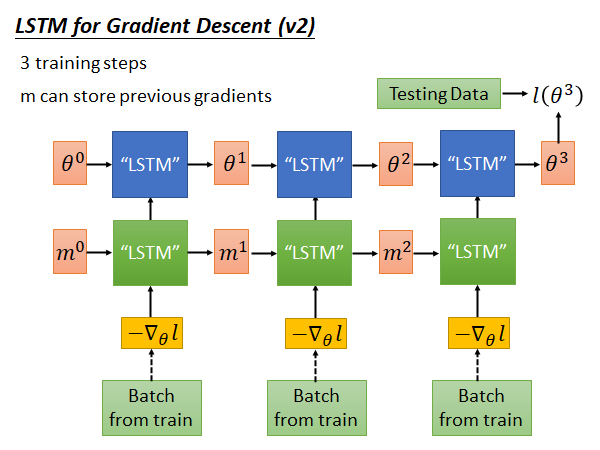## Experimental Result 2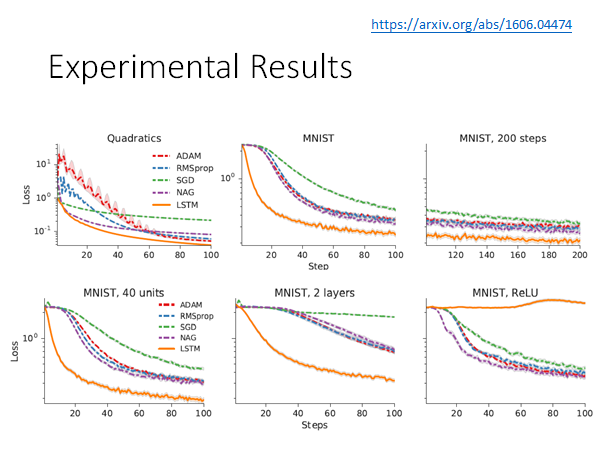https://arxiv.org/abs/1606.04474